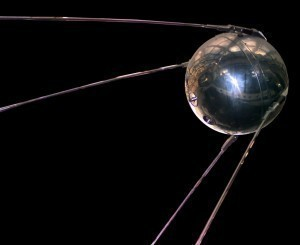# Sputnik Dimensions

The size of the Sputnik was 30.0 m / 98 ft (height)with a diameter of 2.99 m (9.8 ft). Its mass was 267,000 kg (590,000 lbs).

### Sputnik General Characteristics

The payload was 500 kg (1,100 lbs). Another model of the Sputnik called the 8A91 was 31.1 m (102 ft) high. Its mass was 269,300 kg (594,000 lb). At stage 0, the engine was the RD-107 with a thrust of 220,000 lbf (970 kN). The specific impulse was 306 sec with a burn time of 120 seconds. The fuel was LOX/RP-1.

At stage 1, the engine was 1 RD-108 with a thrust of 205,000 lbf (912 kN). The specific impulse was 308 sec. The burn time was 330 seconds. The fuel was LOX/RP-1.

### Specifications and Size of the Sputnik Rocket

At stage number 0 were strap-on boosters (8K71PS-0). The empty mass was 3,400 tons. The thrust was measured at 4 × 99,000 kgf = 396 Mgf (3.89 MN) (vac). The burn time was 120 s (2 min) while the isp was 306 s (3,000 N s/kg).

Its diameter was 2.7 m (8.9 ft) with a span of 2.7 m (8.9 ft). The length was 19 meters (62 ft) with LOX/RP-1 propellants. The engine was the RD-107-8D74PS.

At stage number 1, the gross mass was 94.0 tons with an empty mass of 7.495 tons. The size of the Sputnik rocket gave it a thrust of 99,000 kgf (970 kN). The isp was 308 s (3,020 N s/kg). The diameter was 3 m (9.8 ft) with a burn time of 310 s (5 min 10 s).

The span was 3 m (9.8 ft) with a length of 28 m (92 ft). The LEO payload was 500 kg with a liftoff thrust of 3.89 MN. The engine was RD-108-8D75PS.

On October 4, 1957, the Sputnik rocket was utilized to launch the Sputnik 1. The Sputnik 1 was 83.6 kg (184.3 lbs) with a semi major axis of 6,995.2 km (4,321.8 mi).

### Sputnik 8A91 Technical Data

At stage number 0 the gross mass was 43 tons with an empty mass of 3,400 tons. The isp was 310 s (3,040 N s/kg). The thrust (vac) was 4 × 99,000 kgf = 396 Mgf (3.89 MN).

The size of the Sputnik gave it a burn time of 130 s (2 min 10 s) with a diameter of 2.7 m (8.9 ft). The length was 18 m (62 ft). The propellant was the Lox/RP-1 with the engine RD-107-8D76.

At stage number 1 the empty mass was 7,100 tons. The burn time was 360 s (6 min) with the isp at 315 s (3,090 N s/kg). The thrust was 82,000 kgf (804 kN). The length was 28 m (92 ft). The diameter was 2.99 m (9.8 ft).

The engine was RD-108-8D77 and the propellant was LOX/RP-1. The LEO payload was 1,327 kg (2,925 lb) and had a liftoff thrust of 385,950 kgf (3.784 MN, 850,870).

The size of the Sputnik was striking enough. But more than that, the Sputnik spurned on the race to space and eventually led to the landing on the Moon.